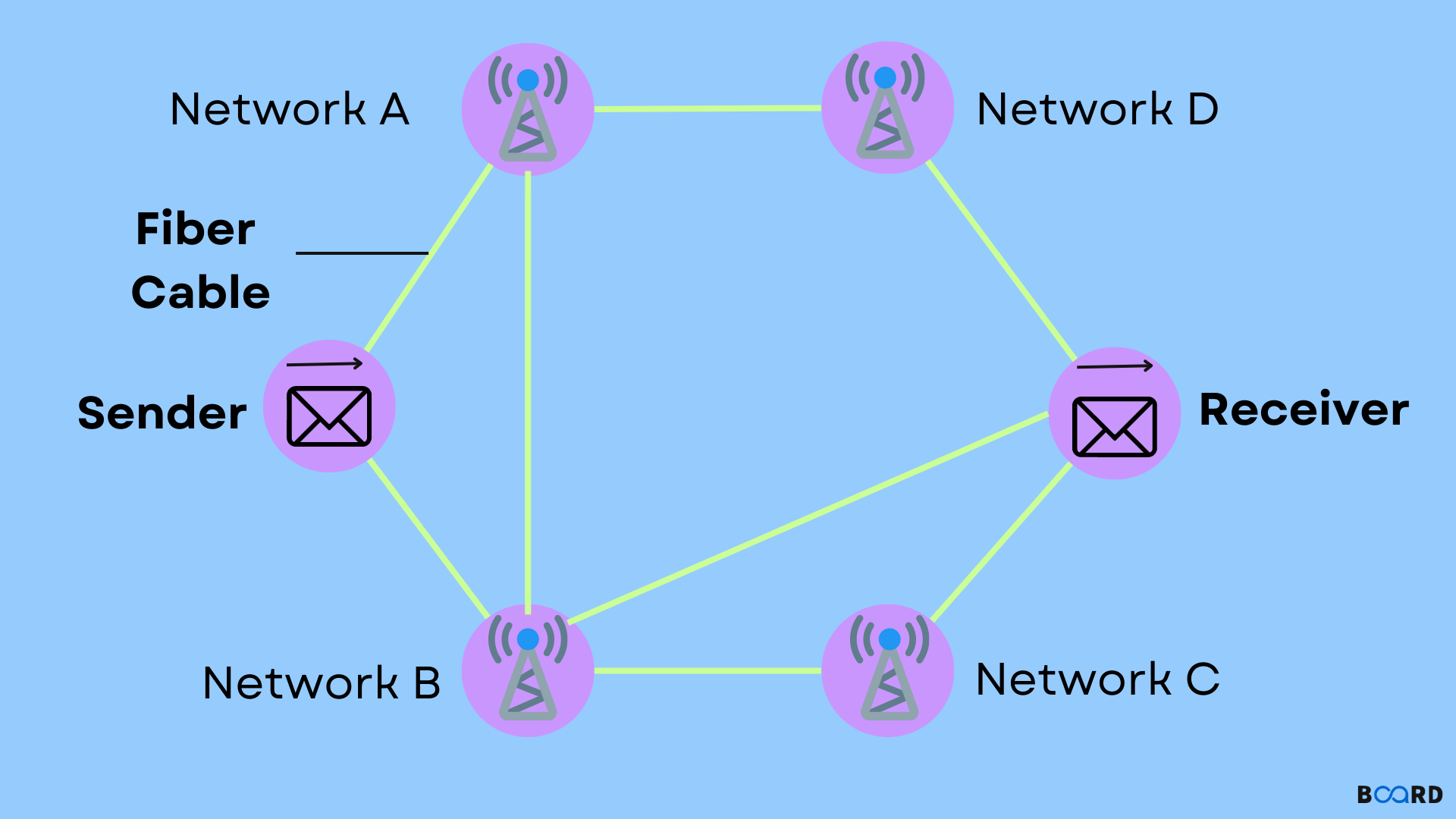# Ford Fulkerson Algorithm (Maximal flow problem)## Overview

In this article, we shall learn about the Ford-Fulkerson algorithm. It is a greedy algorithm for computing the highest possible flow in a graph or network.

## What is Ford-Fulkerson algorithm?

The term “flow network” refers to a network of edges and nodes having a source ‘s’ and a sink ‘t’. Each node other than a and t can send and receive an equal amount of load through it. T can only receive and s can only send.

Have a look at the diagram ahead, to have a better understanding of the algorithm through a flow of fluid inside an interconnection of pipes with varying capacities. Through any pipe only up to a particular amount of fluid will be transferred. Using the Ford-Fulkerson algorithm we determine the amount of fluid that could flow from the s to t.

## Terminologies

### Augmenting Path

The path available in the flow graph.

### Residual Graph

Denotes the flow graph having extra flow possible.

### Residual Capacity

The edge’s remainder capacity after the reduction of the flow from the maximum capacity.

## Working

• Initialize the flow with its value set to zero for each edge.
• So long as there exists an augmenting path between s and t, add the path to the flow.
• Update the residual graph.
• If required, we must also take into consideration the reverse path. Otherwise, we might never find the maximum flow.

Have a look at the diagram ahead to understand the approach explained above:

The flow at each edge is set to zero at the start.

Select any path from source (S) to sink (T), say, the path S -> A -> B -> T

2 (B-T) is the smallest capacity among all of the 3 edges. Now, update the capacity/flow for all the paths.

Now, select some other path, say, S -> D -> C -> T. 3(S-D) is the smallest capacity among all the edges.

Let us now update all the capacities.

Consider the reverse path B -> D too. Now select the path S -> A -> B -> D -> C -> T. 1(D-C) is the smallest residual capacity among all the edges.

Make updates to all the capacities.

We separately consider the capacity for reverse and forward paths. Taking the sum of all the flows: 1+2+3 = 6. This is the highest possible flow in the graph. It must be noticed that if ever the capacity of an edge is full then the path becomes unusable.

## Implementation

 #include "iostream"#include "vector"#include "list"#include "limits.h"#include "stack"#include "unordered_map"#include "unordered_set"#include "set"#include "map"#include "math.h"#include "algorithm"#include "queue"#include "functional"#include "numeric"#include "iomanip"#include "bitset"using namespace std;bool bfs(const vector>&rg, int s, int t, vector&par){    int n=rg.size();    vectorvis(n);    queue q;    q.push(s);    vis[s] = 1, par[s] = -1;        while(!q.empty()) {        int u = q.front();        q.pop();        for (int v = 0; v < n; v++) {        if(!vis[v] && rg[u][v]>0) {            q.push(v);            par[v] = u, vis[v] = 1;        }    }  }  return vis[t];}int fordFulkerson(const vector>&g, int s, int t) {  int u, v, n=g.size(), maxFlow=0;  auto rg=g;  vectorpar(n);  while(bfs(rg, s, t, par)){    int pathFlow = INT_MAX;    for(v = t; v != s; v = par[v]) {        u = par[v];        pathFlow = min(pathFlow, rg[u][v]);    }        for(v = t; v != s; v = par[v]) {        u = par[v];        rg[u][v] -= pathFlow;        rg[v][u] += pathFlow;    }        maxFlow += pathFlow;  }  return maxFlow;}int main(){    vector>g={{0, 8, 0, 0, 3, 0},             {0, 0, 9, 0, 0, 0},             {0, 0, 0, 0, 7, 2},             {0, 0, 0, 0, 0, 5},             {0, 0, 7, 4, 0, 0},             {0, 0, 0, 0, 0, 0}};    cout<<"The max flow is:"<

Output

 The max flow is:6
write your code here: Coding Playground